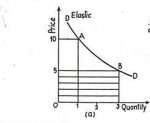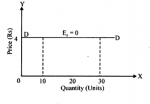# ISC ECONOMICS 12 Elasticity of Demand MCQs with Solved Answers

## (Question 1 t0 5)

1. Demand curve (DD) is a horizontal straight line parallel to X-axis in case of..
(a) Perfectly Elastic Demand
(b) Perfectly Inelastic Demand
(c) Unitary Elastic Demand
(d) Highly Elastic Demand
(e) Less Elastic Demand

2. Demand curve (DD) is a vertical straight line parallel to Y-axis in case of..
(a) Perfectly Elastic Demand
(b) Perfectly Inelastic Demand
(c) Unitary Elastic Demand
(d) Highly Elastic Demand
(e) Less Elastic Demand

3. Demand curve (DD) is a rectangular hyperbola in case of..
(a) Perfectly Elastic Demand
(b) Perfectly Inelastic Demand
(c) Unitary Elastic Demand
(d) Highly Elastic Demand
(e) Less Elastic Demand

4. Demand curve (DD) is flatter and its slope is inclined more towards X-axis, in case of..
(a) Perfectly Elastic Demand
(b) Perfectly Inelastic Demand
(c) Unitary Elastic Demand
(d) Highly Elastic Demand

5. Demand curve (DD) is steeper and its slope is inclined more towards Y-axis, in case of..
(a) Perfectly Elastic Demand
(b) Perfectly Inelastic Demand
(c) Unitary Elastic Demand
(d) Highly Elastic Demand
(e) Less Elastic Demand

ISC Commerce 12 Planning MCQs with Solved Answers

Note: Answers are given below at the end.

## (Question 6 t0 10)

6. When A slight or no change in the price leads to infinite changes in the quantity demanded. Demand of a commodity is said to be ……..

(a) Perfectly Elastic Demand
(b) Perfectly Inelastic Demand
(c) Unitary Elastic Demand
(d) Highly Elastic Demand

7. when, Demand of a commodity does not change at all irrespective of any change in its price.Demand of a commodity is said to be ……..

(a) Perfectly Elastic Demand
(b) Perfectly Inelastic Demand
(c) Unitary Elastic Demand
(d) Highly Elastic Demand

8. When the percentage change in Demand of a commodity is equal to the percentage change in price. Demand of a commodity is said to be ……..

(a) Perfectly Elastic Demand
(b) Perfectly Inelastic Demand
(c) Unitary Elastic Demand
(d) Highly Elastic Demand

9. When percentage change in Demand of a commodity is more than the percentage change in its price. Demand of a commodity is said to be ……..

(a) Perfectly Elastic Demand
(b) Perfectly Inelastic Demand
(c) Unitary Elastic Demand
(d) Highly Elastic Demand

10.When percentage change in Demand of a commodity is less than the percentage change in its price. Demand of a commodity is said to be ……..

(a) Less Elastic Demand  (Ed <1)
(b) Perfectly Inelastic Demand
(c) Unitary Elastic Demand (Ed =1)
(d) Highly Elastic Demand (Ed >1)

ISC Commerce 12 Sources of Finance MCQs with Solved Answers

Note: Answers are given below at the end.

## (Question 11 t0 15)

11. A negative sign with coefficient of price elasticity of demand denotes?
(a) Direct relation between price of the commodity and quantity demanded.
(b) No relation between price of the commodity and quantity demanded.
(c) Inverse relation between price of the commodity and quantity demanded.
(d) None of these

12. Demand for a good in less elastic when:
(a) Percentage change in price > Percentage change in quantity demand
(b) Percentage change in quantity Deemanded > Percentage change in price
(c) Percentage change in price = Percentage change in quantity demanded
(d) Demand remains same even with change in price

13. If the price elasticity of demand for a commodity is less than unity, a decrease in price would result in:
(a) Proportionately less increase in the quantity demanded
(b) Proportionately more increase in the quantity demanded
(c) Increase in total expenditure on the product
(d) None of these

14. Which of the following will have elasatic demand
(a) Matchbox
(b) ISC Textbook
(c) Medicine
(d) Air Conditioners

15.  Which one of the following statement is incorrect
(a) Higher numerical value of elasticity indicates larger effect of a price changes on the quantity demanded.
(b) Elasticity of demand can vary only between -1 and +1
(c) The demand curves for all commodities which have unitary elastic demand will be a rectangular hyperbola
(d) Elasticity of demand establishes a quantitative relationship between quantity and demanded of a commodity and its price, while other factors remain constant

ISC Economics 12 Demand MCQs With Solved Answer

Note: Answers are given below at the end.

## (Question 16 t0 20)

16. The following diagram represents ______ elastic demand for commodity X(a) Less
(b) Highly
(c) Unitary
(d) Perfectly

17. The following diagram represents ______ elastic demand for commodity X(a) Less
(b) Highly
(c) Unitary
(d) Perfectly

18. The following diagram represents ______ elastic demand for commodity X(a) Less
(b) Highly
(c) Unitary
(d) Perfectly

19. As price of a commodity increases from ₹ 40 per unit to ₹ 50 per unit, demand falls from 200 units to 100 units. What will be the elasticity of demand

(a) (-)2
(b) (-)1
(c) 0
(d) ∞

20. With increase in price of Pizza by 22%, its demand falls by 25%. This indicates that demand for Pizza is:

(a) Elastic
(b) Inelastic
(c) Unitary Elastic
(d) Perfectly Elastic

Note: Answers are given below at the end.

## Answer – Question Number 1To 5

3.Answer – (c) Unitary Elastic Demand

4.Answer – (d) Highly Elastic Demand

5.Answer – (e) Less Elastic Demand

## Answer – Question Number 6 To 10

6.Answer – (a) Perfectly Elastic Demand

9.Answer – (d) Highly Elastic Demand

10.Answer – (a) Less Elastic Demand

## Answer – Question Number 11 To 15

11.Answer – (c) Inverse relation between price of the commodity and quantity demanded.

12.Answer – (a) Percentage change in price > Percentage change in quantity demand

13.Answer – (a) Proportionately less increase in the quantity demanded

15.Answer – (b) Elasticity of demand can vary only between -1 and +1

## Answer – Question Number 16 To 20

### 1 thought on “ISC ECONOMICS 12 Elasticity of Demand MCQs with Solved Answers”

1.How come Firefox displays a weird character when it should show a word?